Function Repository Resource:

# CrossTabulate

Compute the contingency table for a two- or three- column dataset or array

Contributed by: Anton Antonov
 ResourceFunction["CrossTabulate"][data] finds the contingency table for the Dataset or array data.

## Details and Options

ResourceFunction["CrossTabulate"] works on two dimensional full arrays with two or three columns, or on datasets that can be represented in that way.
If present, the third column is expected to be numerical.
If the argument has two columns, the computed contingency values are co-occurance counts for each unique pair of values of the first and second columns.
If the argument has three columns, the computed contingency values are sums of the third column values for each unique pair of values of the first and second columns.

## Examples

### Basic Examples (2)

Here is an array of random integer-word pairs:

 In:=Out=Compute the contingency table:

 In:=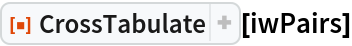Out=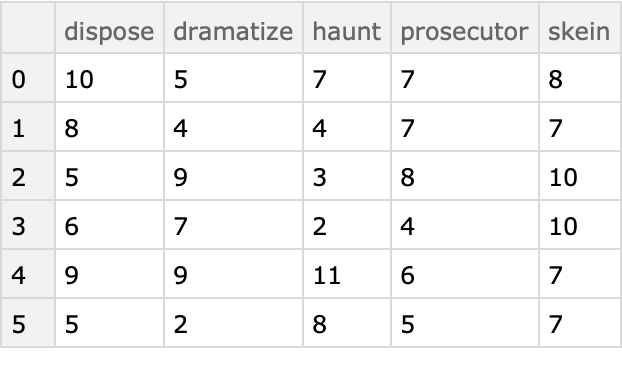Here is a Dataset, the first two columns of which are categorical columns and the third column which is numeric:

 In:=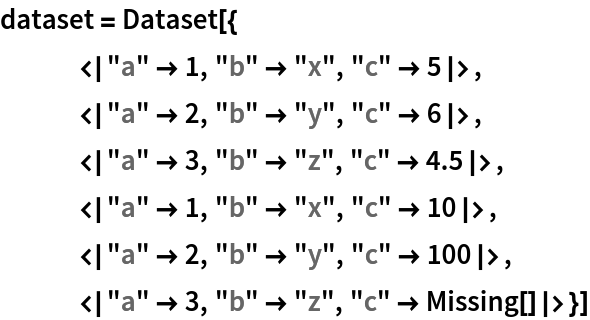Out=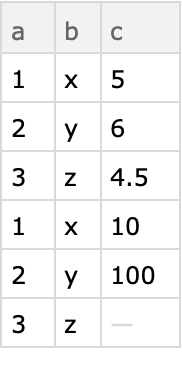Compute the contingency table:

 In:=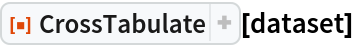Out=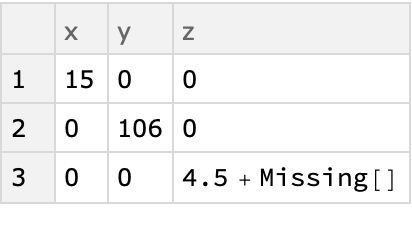### Scope (5)

#### Result representation (1)

For large contingency tables instead of using Dataset it is faster and more convenient to use sparse arrays. That is specified with the option “Sparse”:

 In:=Out=In:=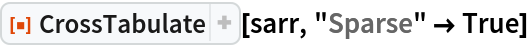Out=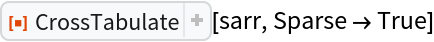#### Using a third, numerical column (3)

Here is a full array with three columns:

 In:=Out=Compute the contingency table of the co-occurrences of each letter and with each word found by cross tabulating over the first two columns only:

 In:=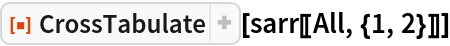Out=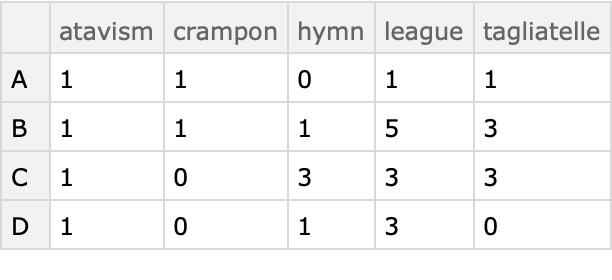Here the cross tabulation uses the third column -- for each unique letter-word pair the corresponding values of the third column are added:

 In:=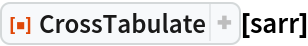Out=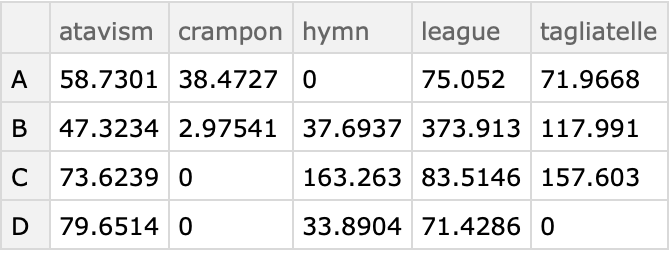#### Missing values (1)

If any of the columns have missing values they are shown in the contingency table:

 In:=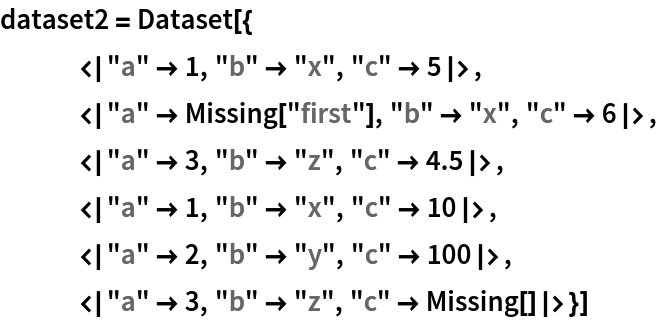Out=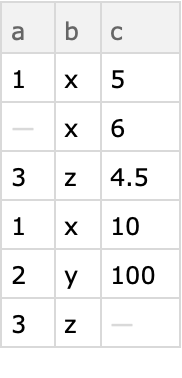In:=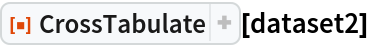Out=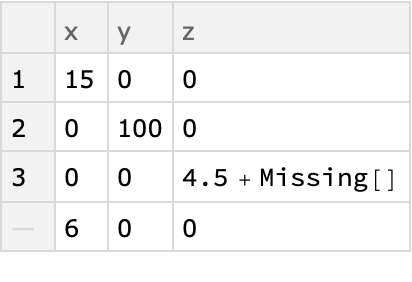### Options (2)

#### Sparse (2)

The result of CrossTabulate is a Dataset by default. With the option setting "Sparse"True the result is an Association with three elements: a sparse matrix with the contingency values, row names, and column names.

Here is an example:

 In:=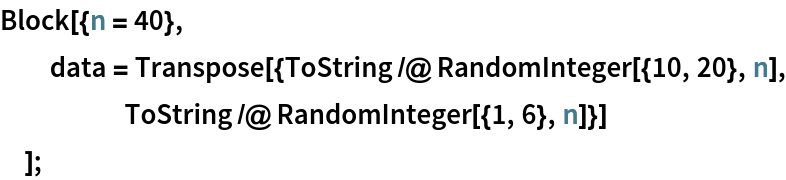In:=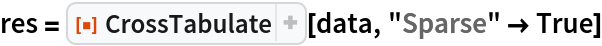Out=Using MatrixForm we can visualize the result:

 In:=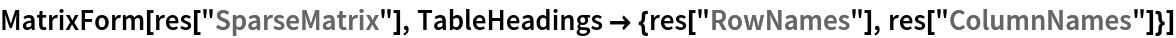Out=### Applications (13)

#### Data study (5)

Take the Titanic dataset:

 In:=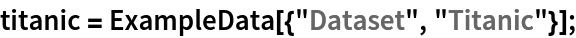Find how many males and females are in each passenger class:

 In:=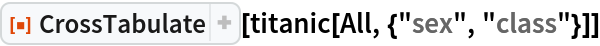Out=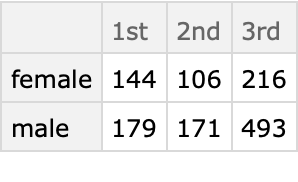Find how many males and females survived:

 In:=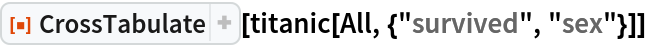Out=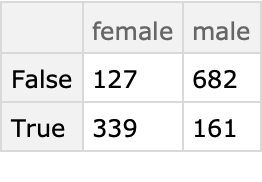Find the aggregated ages of the class-sex breakdown:

 In:=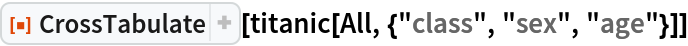Out=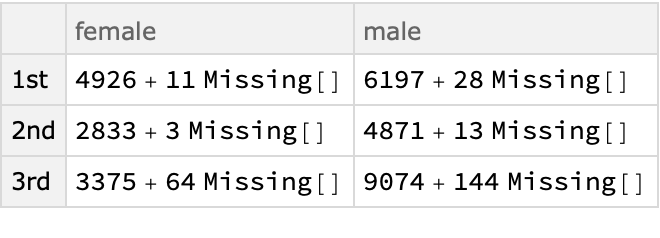Here is a function to plot sparse contingency tables:

 In:=In:=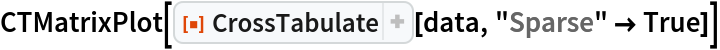Out=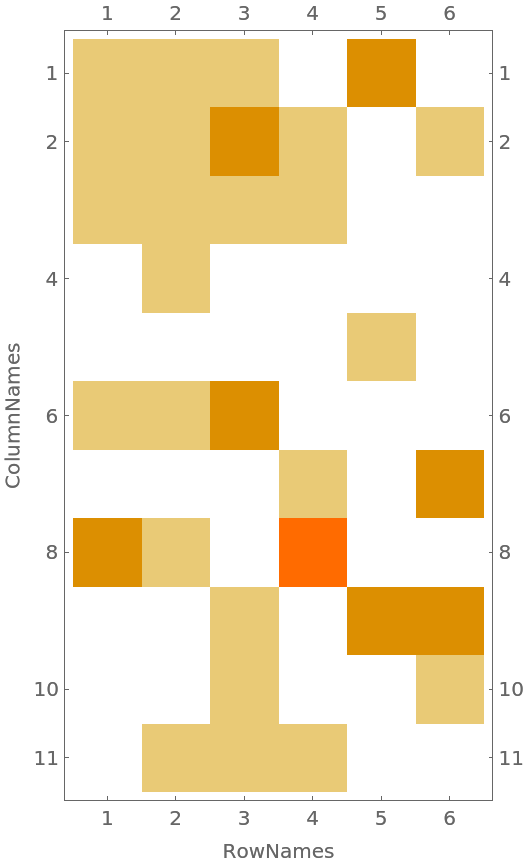#### Word-sentiment analysis of movie reviews (8)

 In:=Out=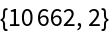For each movie review we make a list of word-sentiment pairs and then join them into one big list:

 In:=Out=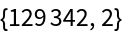Here is a sample:

 In:=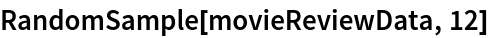Out=Here we find the word-sentiment contingency table as a sparse matrix in order to plot it below:

 In:=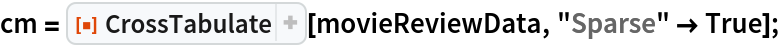Here is a function to plot sparse contingency tables:

 In:=Plot the contingency table:

 In:=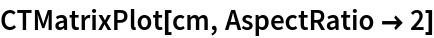Out=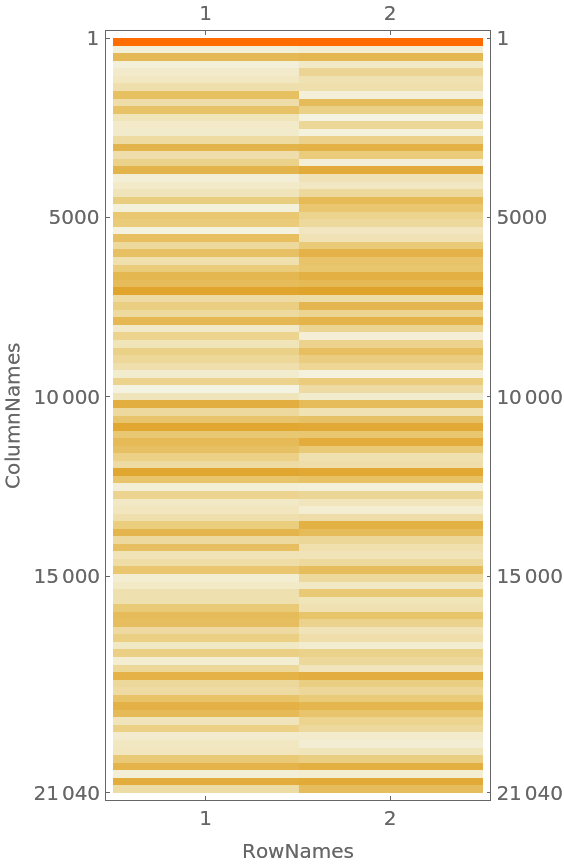Find the contingency table Dataset:

 In:=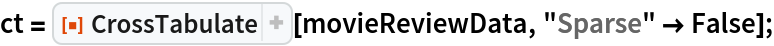Show the most prominent words for negative reviews:

 In:=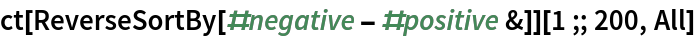Out=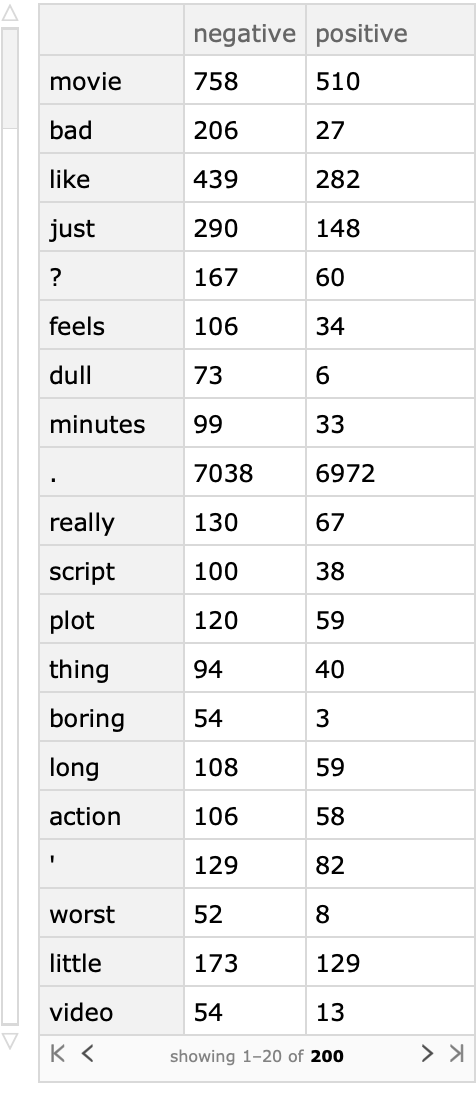### Properties and Relations (6)

#### Tally and GroupBy (5)

The functionality of CrossTabulate can be emulated with Tally or GroupBy.

Here is a contingency matrix of a two column array:

 In:=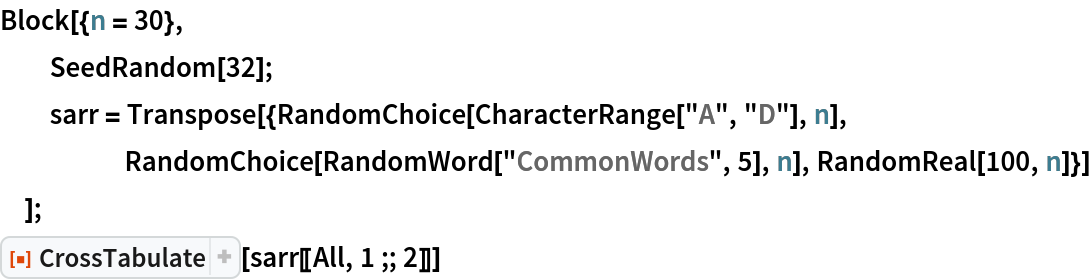Out=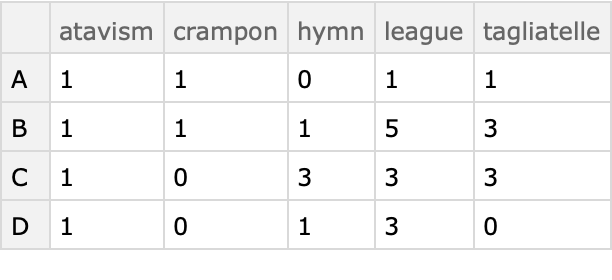Obtain the contingency value triplets using Tally:

 In:=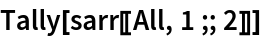Out=Obtain the contingency values rules using GroupBy:

 In:=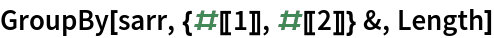Out=GroupBy generalizes better than Tally -- we can use GroupBy to get the contingency values for three column data:

 In:=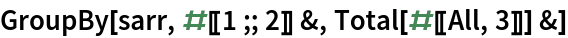Out=Find the corresponding result of CrossTabulate:

 In:=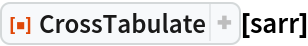Out=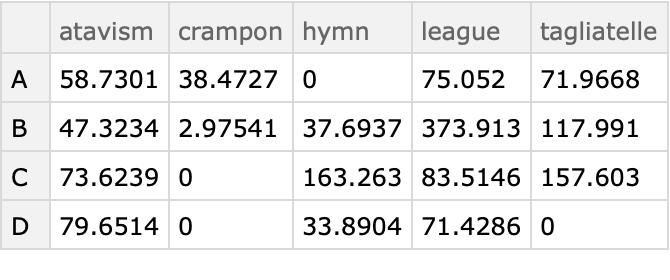#### Conversion sparse results into datasets (1)

Convert the Association obtained with the option setting "Sparse"True into a Dataset:

 In:=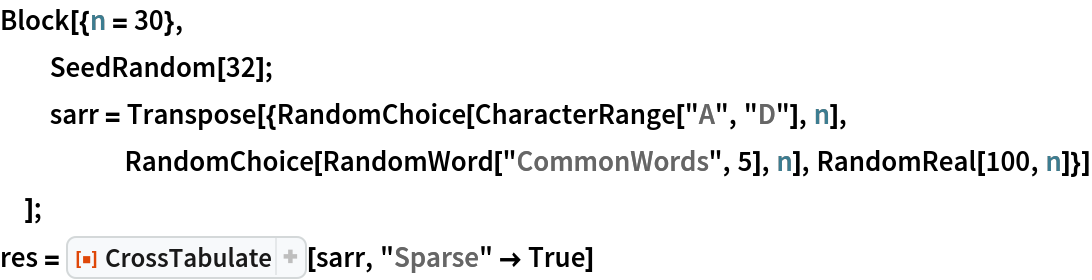Out=In:=Out=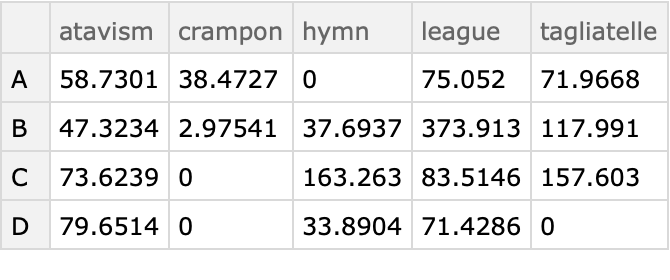### Possible Issues (4)

If the second variable is numerical or has missing values the resulting Dataset would not have a tabular form:

 In:=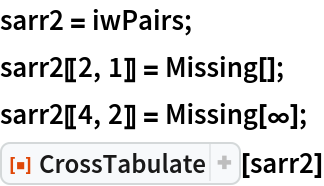Out=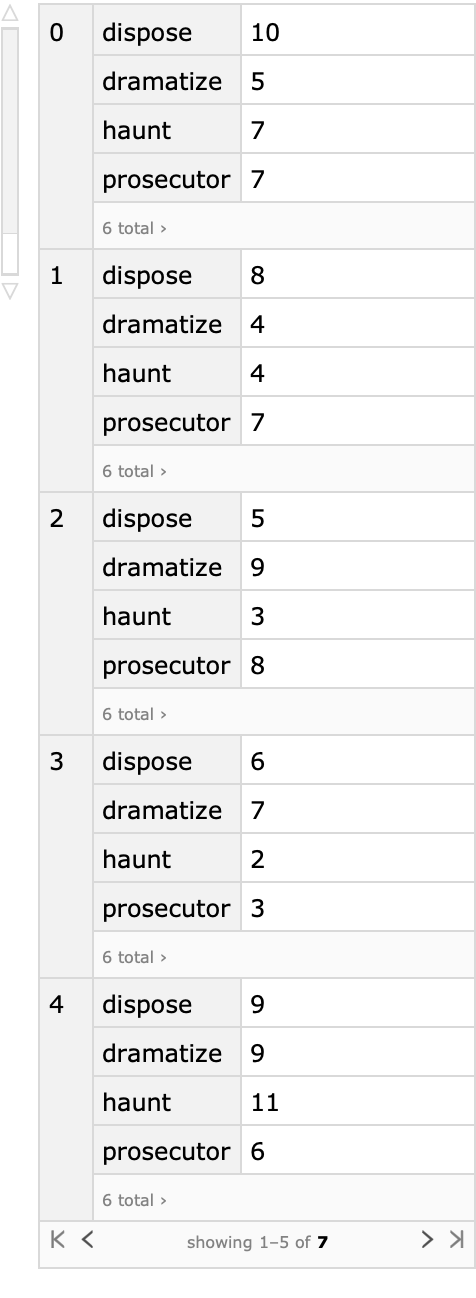One way to get a tabular form is to replace Missing[___] with a string:

 In:=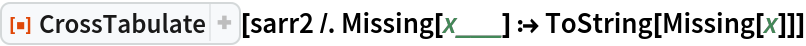Out=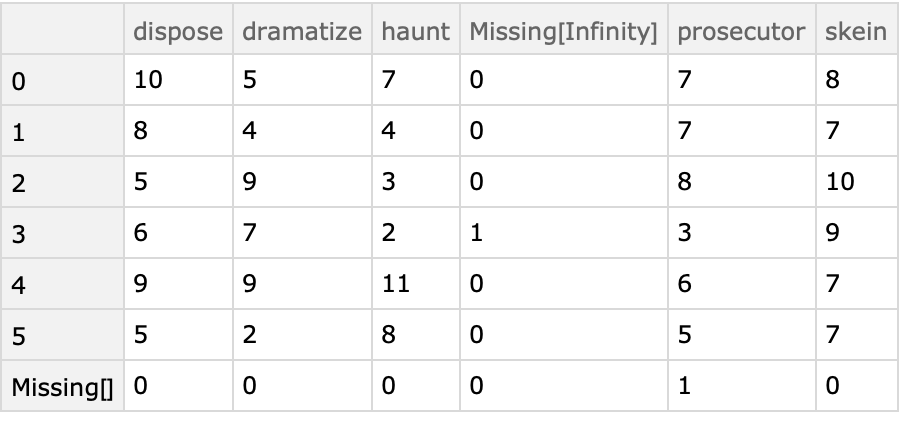Find the co-occurrence of the integers [1,3] in a list of random integer pairs:

 In:=Out=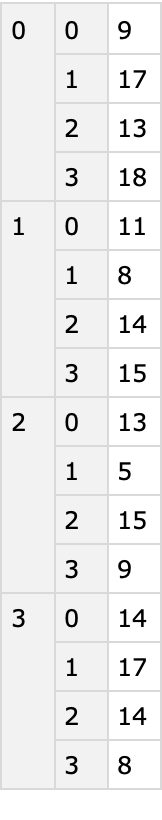Again, replacing the integer values with strings produces tabular form:

 In:=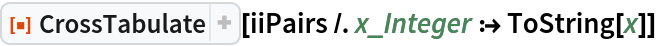Out=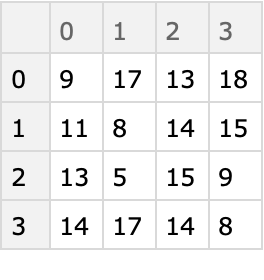### Neat Examples (1)

Here is a grid of contingency tables showing various breakdown perspectives of the Titanic data:

 In:=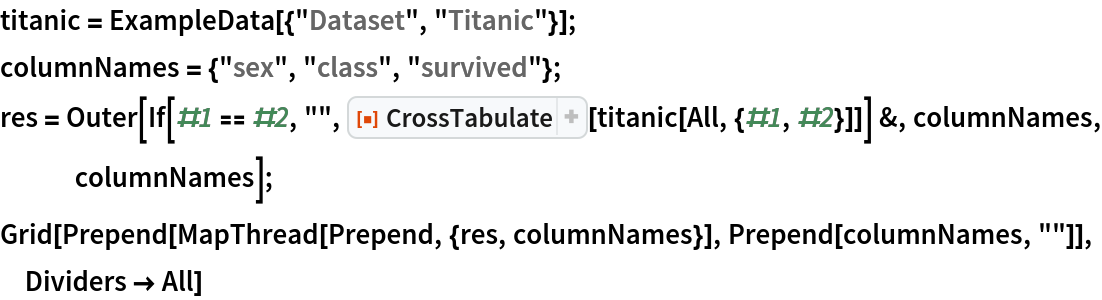Out=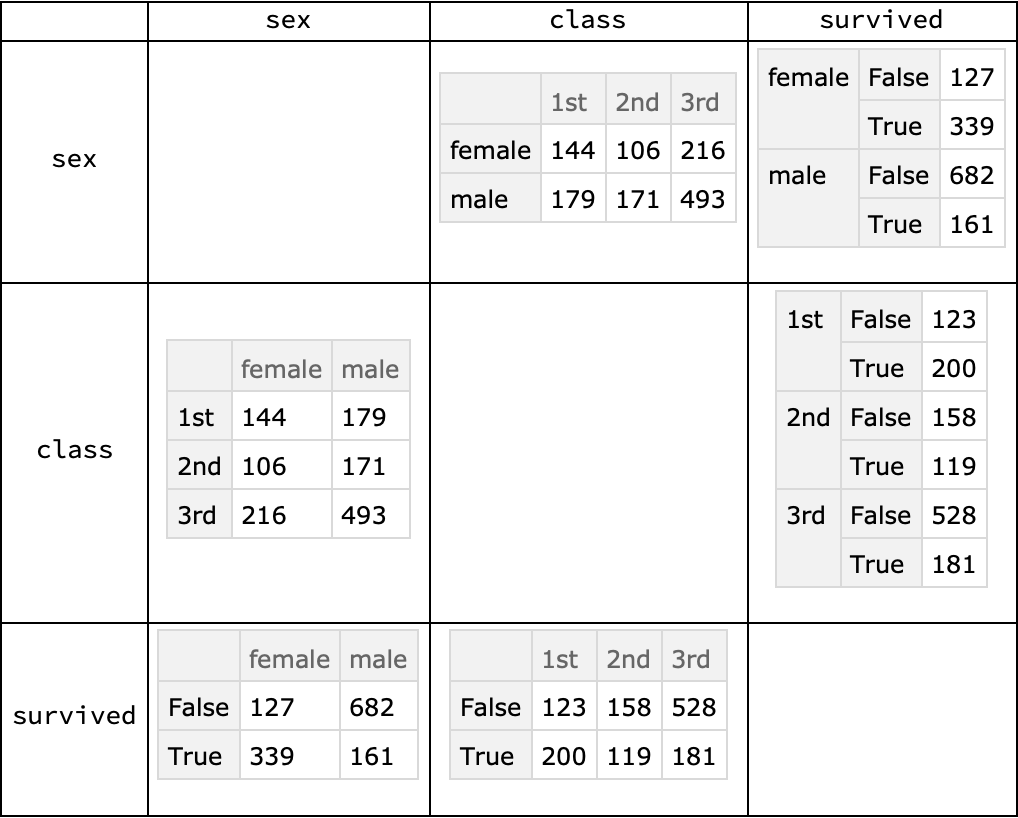Anton Antonov

## Version History

• 1.0.0 – 17 October 2019

## Author Notes

The function CrossTabulate corresponds to R’s function xtabs.